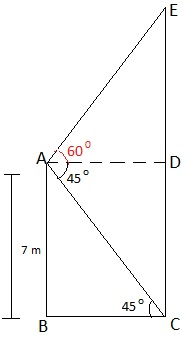Guru

# From the top of a 7 m high building, the angle of elevation of the top of a cable tower is 60° and the angle of depression of its foot is 45°. Determine the height of the tower. Q.12

• 0

I Want simple and easy way to solve this tough question from class 10th ncert math of chapter “Some applications of Trigonometry ncert” of exercise 9.1 question no.12. Sir please give me the easiest way to solve this problem From the top of a 7 m high building, the angle of elevation of the top of a cable tower is 60° and the angle of depression of its foot is 45°. Determine the height of the tower.

Share

1. Let AB be the building of height 7 m and EC be the height of the tower.

A is the point from where elevation of tower is 60° and the angle of depression of its foot is 45°.

EC = DE + CD

Also, CD = AB = 7 m. and BC = AD

To Find: EC = Height of the tower

Design a figure based on given instructions:In right ΔABC,

tan 45° = AB/BC

1= 7/BC

BC = 7